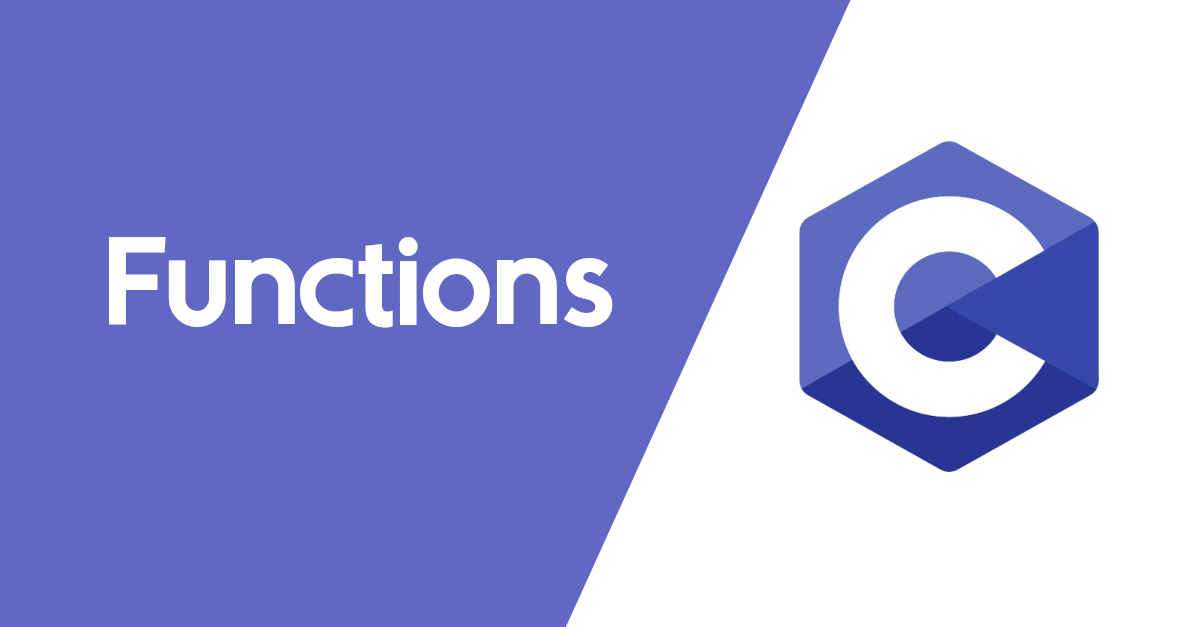What do you understand by function? We see in mathematics, function can be defined as y = f(x), where function of x is assigned to y.

Here x and y both are variable. But x is independent variable and y is dependent (on x) variable.

For any value of x this function returns at least one value for y.

Example: If `y = x2` be a functional statement and the let the value of x is 2 then the function will return 4 as the value of y.

Oh, x in the function is called argument.

Exactly in C Programming language, function works as the above.

In C program, we have to define the name, data type of function and argument as like variable.

Suppose we want to make a function that will take integer number as argument and return the square of the argument, about which function has been discussed just before.

So we have to first the data type of function as int and name it.
``````int square(int x)
{
int y;
y = x * x;
return (y);
}``````
Observation:
• Defining integer type of the function means, this functional will return result as integer.
• Defining integer type of argument means, this function can only input integer number.
• In C, argument must be within parentheses.
• The process of this function enclosed by curly braces. First curly brace to start the function and second to end.
• Int y to declaration variable y as local variable.
• `y = x * x;` is the functional calculation.
• `return(y);` will return the value of y after calculation as output of this function.
Passing more than one argument.
Multiple returns.

#### Types of Function in C

Functions in computer science are two types: Built-in function and user defined function.

1. Built-in Function: This function is built by the author of programming language to make easy to access program. We can call it ready made function.

Some example of built-in function:

Main function, print function, scan function are the great example of built-in function.

2. User-Defined Function:
What is user defined function?
User defined function is a type of function that is built by user.
That means, programming language like C allows to create new function.

Elements of user defined function:

• Prototype
• Function Definition
◦ Function Data Type
◦ Function Name
◦ Function Parameter
◦ Function Operation
◦ Function Return
• Calling Function from Main Function

Example of User-Defined Function:
``````#include <stdio.h>
int sum(int a, int b); //Prototype
int main() //Main function
{

int result;
result = sum(10,5); //Calling/caller Function

printf("%d\n", x);
return 0;
}

int sum(int a, int b) //Function definition
{
return (a+b); //Function return the sum of a and b.
}``````
Prototype: Prototype in function of C is the declaration of user-defined function to use that is declared before main function.
Example: `int sum(int a, int b);`

Function Definition: Function definition is a process to define the function how will it works, what will take input, what will return to main function, which data type parameter, return type will permit it. It is the main part of user-defined function.

Function Data Type:
The data type of function defines what kind of data this function will return.

If we define as `int, float, double` it will return integer, float, double value to main function respectively.

Function Name: The name of function must be declared before using function like variable. The rules of function name is similar as variable declaration in C.

Function Parameter: Parameter of function is the resources for which input function can return output by processing. Parameters are defined within parentheses after function name. Multiple parameters are separated by comma.

• In function declaration and definition parameters should be same name but must be same of data type.
• In function definition, operation must be organized with parameter of its.
• In main function, use declared variable as parameter in calling function.

Function Operation: Function operation is the process to make output.

Function Return: To make output, user defined function returns something to main function by calling function.

Calling Function from Main Function:It is not enough to define a function in program (for any programming language). We have to call it main function. Probably you know that, every C program must have a main function. It is called main function because, whatever we want to do through program, we have to do from main function. It is the root of program. In interpretation, compiler actually compiles base on main function statement-by-statement. If statement be a function that is defined outer from main function, then the compiler calls the defined function.

Caller Function:
The function is defined within main function is called caller function. Caller function contains initialized value/variable as arguments.
Here `sum(10,5);` is the caller function.
Called Function: The defined function outer the main function is known as called function.
Here -
``````int sum(int a, int b) //Function definition
{
return (a+b); //Function return the sum of a and b.
}``````
is called function.